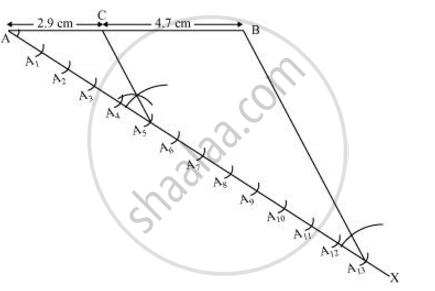Share

# Draw a line segment of length 7.6 cm and divide it in the ratio 5:8. Measure the two parts. Give the justification of the construction. - CBSE Class 10 - Mathematics

#### Question

Draw a line segment of length 7.6 cm and divide it in the ratio 5:8. Measure the two parts. Give the justification of the construction.

#### Solution

A line segment of length 7.6 cm can be divided in the ratio of 5:8 as follows.

Step 1 Draw line segment AB of 7.6 cm and draw a ray AX making an acute angle with line segment AB.

Step 2 Locate 13 (= 5 + 8) points, A1, A2, A3, A…….. A13, on AX such that AA1 = A1A= A2A3 and so on.

Step 3 Join BA13.

Step 4 Through the point A5, draw a line parallel to BA13 (by making an angle equal to ∠AA13B) at A5intersecting AB at point C.

C is the point dividing line segment AB of 7.6 cm in the required ratio of 5:8.

The lengths of AC and CB can be measured. It comes out to 2.9 cm and 4.7 cm respectively.Justification

The construction can be justified by proving that

(AC)/(CB) = 5/8

By construction, we have A5C || A13B. By applying Basic proportionality theorem for the triangle AA13B, we obtain

(AC)/(CB) =(

From the figure, it can be observed that AA5 and A5A13 contain 5 and 8 equal divisions of line segments respectively

:. (

On comparing equations (1) and (2), we obtain

(AC)/(CB) =  5/8

This justifies the construction

Is there an error in this question or solution?

#### APPEARS IN

NCERT Solution for Mathematics Textbook for Class 10 (2019 to Current)
Chapter 11: Constructions
Ex. 11.10 | Q: 1 | Page no. 219
Solution Draw a line segment of length 7.6 cm and divide it in the ratio 5:8. Measure the two parts. Give the justification of the construction. Concept: Division of a Line Segment.
S